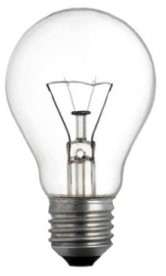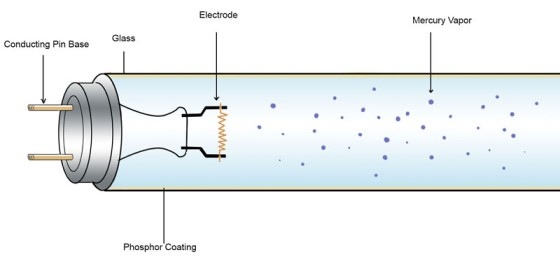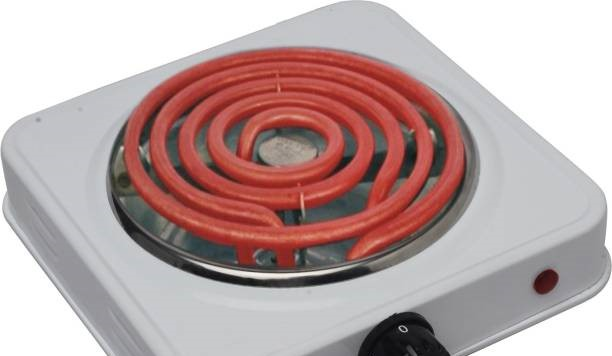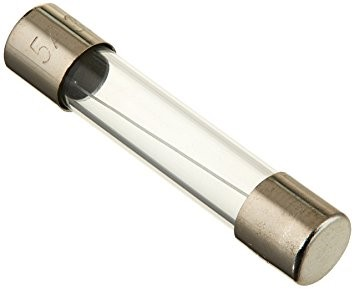# Heating effect of an electric current

### Guest Account

Attempt Form Three Physics Questions
Guest Account

# Heating effect of an electric current

- When current flows, electrical energy is transformed into other forms of energy i.e. light, mechanical and chemical changes.

### Factors affecting electrical heating

Energy dissipated by current or work done as current flows depends on,
1. Current
2. Resistance
3. Time
This formula summarizes these factors as, E = I2 R t, E = I V t or E = V2t / R
Example 1
An iron box has a resistance coil of 30 Ω and takes a current of 10 A. Calculate the heat in kJ developed in 1 minute.
Solution

E = I2 R t = 102 × 30 × 60 = 18 × 104 = 180 kJ

Example 2
A heating coil providing 3,600 J/min is required when the p.d across it is 24 V. Calculate the length of the wire making the coil given that its cross-sectional area is 1 × 10-7 m2 and resistivity 1 × 10-6 Ω m.
Solution

E = P t hence P = E / t = 3,600 / 60 = 60 W
P = V2/ R therefore R = (24 × 24) / 60 = 9.6 &ohms;
R = ρI/ A, I = (RA) / ρ = (9.6 × 1 × 10-7) / 1 × 10-6 = 0.96 m

### Electrical energy and power

In summary, electrical power consumed by an electrical appliance is given by;
P = V I
P = I2R
P = V2/ R
The SI unit for power is the watt (W)
1 W = 1 J/s and 1kW = 1,000 W.

Example 3
What is the maximum number of 100 W bulbs which can be safely run from a 240 V source supplying a current of 5 A?
Solution

Let the maximum number of bulbs be 'n'. Then 240 × 5 = 100 n
So 'n' = (240 × 5) / 100 = 12 bulbs.

Example 4
An electric light bulb has a filament of resistance 470 Ω. The leads connecting the bulb to the 240 V mains have a total resistance of 10 Ω. Find the power dissipated in the bulb and in the leads.
Solution

Req = 470 + 10 = 480 Ω, therefore I = 240 / 480 = 0.5 A.
Hence power dissipated = I2 R = (0.5)2 × 470 = 117.5 W (bulb alone)
For the leads alone, R = 10 Ω and I = 0.5 A
Therefore power dissipated = (0.5)2 × 10 = 2.5 W.

### Applications of heating of electrical current

1. Filament lamp – the filament is made up of tungsten, a metal with high melting point (3.400 0C). It is enclosed in a glass bulb with air removed and argon or nitrogen injected to avoid oxidation. This extends the life of the filament.2. Fluorescent lamps – when the lamp is switched on, the mercury vapour emits ultra violet radiation making the powder in the tube fluoresce i.e. emit light. Different powders emit different colours.3. Electrical heating – electrical fires, cookers e.tc. their elements are made up nichrome ( alloy of nickel and chromium) which is not oxidized easily when it turns red hot.4. Fuse – this is a short length of wire of a material with low melting point (often thinned copper) which melts when current through it exceeds a certain value. They are used to avoid overloading.